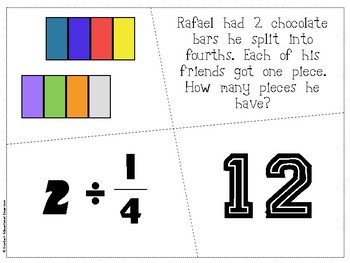# Division with Fractions BUNDLESubject
Resource Type
Format
Zip (3 MB|24 pages)
Standards
\$3.00
Bundle
List Price:
\$3.50
You Save:
\$0.50
\$3.00
Bundle
List Price:
\$3.50
You Save:
\$0.50

### Description

Included are TWO sets of division with fractions puzzles. Each set of puzzles includes 12 puzzles (total of 24) that help students understand the visual, mathematical, and applied concepts of fraction with division.

Included are Whole Numbers / Fractions and Fractions / Whole Numbers

Because these puzzles are self correcting, they are perfect for centers, math review stations, and prep for assessments.

CCSS: 5.NF.B.3; 5.NF.B.7

Total Pages
24 pages
N/A
Teaching Duration
N/A
Report this Resource to TpT
Reported resources will be reviewed by our team. Report this resource to let us know if this resource violates TpT’s content guidelines.

### Standards

to see state-specific standards (only available in the US).
Solve real world problems involving division of unit fractions by non-zero whole numbers and division of whole numbers by unit fractions, e.g., by using visual fraction models and equations to represent the problem. For example, how much chocolate will each person get if 3 people share 1/2 lb of chocolate equally? How many 1/3-cup servings are in 2 cups of raisins?
Interpret division of a whole number by a unit fraction, and compute such quotients. For example, create a story context for 4 ÷ (1/5), and use a visual fraction model to show the quotient. Use the relationship between multiplication and division to explain that 4 ÷ (1/5) = 20 because 20 × (1/5) = 4.
Interpret division of a unit fraction by a non-zero whole number, and compute such quotients. For example, create a story context for (1/3) ÷ 4, and use a visual fraction model to show the quotient. Use the relationship between multiplication and division to explain that (1/3) ÷ 4 = 1/12 because (1/12) × 4 = 1/3.
Apply and extend previous understandings of division to divide unit fractions by whole numbers and whole numbers by unit fractions.
Interpret a fraction as division of the numerator by the denominator (𝘢/𝘣 = 𝘢 ÷ 𝘣). Solve word problems involving division of whole numbers leading to answers in the form of fractions or mixed numbers, e.g., by using visual fraction models or equations to represent the problem. For example, interpret 3/4 as the result of dividing 3 by 4, noting that 3/4 multiplied by 4 equals 3, and that when 3 wholes are shared equally among 4 people each person has a share of size 3/4. If 9 people want to share a 50-pound sack of rice equally by weight, how many pounds of rice should each person get? Between what two whole numbers does your answer lie?